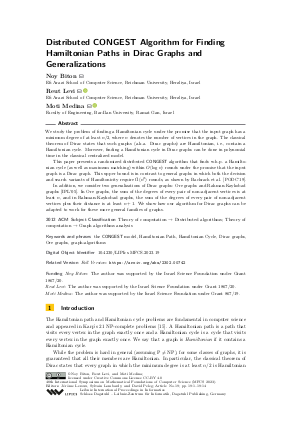Document# Distributed CONGEST Algorithm for Finding Hamiltonian Paths in Dirac Graphs and Generalizations

### Authors Noy Biton, Reut Levi, Moti Medina## File

LIPIcs.MFCS.2023.19.pdf
• Filesize: 0.9 MB
• 14 pages

## Cite As

Noy Biton, Reut Levi, and Moti Medina. Distributed CONGEST Algorithm for Finding Hamiltonian Paths in Dirac Graphs and Generalizations. In 48th International Symposium on Mathematical Foundations of Computer Science (MFCS 2023). Leibniz International Proceedings in Informatics (LIPIcs), Volume 272, pp. 19:1-19:14, Schloss Dagstuhl - Leibniz-Zentrum für Informatik (2023)
https://doi.org/10.4230/LIPIcs.MFCS.2023.19

## Abstract

We study the problem of finding a Hamiltonian cycle under the promise that the input graph has a minimum degree of at least n/2, where n denotes the number of vertices in the graph. The classical theorem of Dirac states that such graphs (a.k.a. Dirac graphs) are Hamiltonian, i.e., contain a Hamiltonian cycle. Moreover, finding a Hamiltonian cycle in Dirac graphs can be done in polynomial time in the classical centralized model. This paper presents a randomized distributed CONGEST algorithm that finds w.h.p. a Hamiltonian cycle (as well as maximum matching) within O(log n) rounds under the promise that the input graph is a Dirac graph. This upper bound is in contrast to general graphs in which both the decision and search variants of Hamiltonicity require Ω̃(n²) rounds, as shown by Bachrach et al. [PODC'19]. In addition, we consider two generalizations of Dirac graphs: Ore graphs and Rahman-Kaykobad graphs [IPL'05]. In Ore graphs, the sum of the degrees of every pair of non-adjacent vertices is at least n, and in Rahman-Kaykobad graphs, the sum of the degrees of every pair of non-adjacent vertices plus their distance is at least n+1. We show how our algorithm for Dirac graphs can be adapted to work for these more general families of graphs.

## Subject Classification

##### ACM Subject Classification
• Theory of computation → Distributed algorithms
• Theory of computation → Graph algorithms analysis
##### Keywords
• the CONGEST model
• Hamiltonian Path
• Hamiltonian Cycle
• Dirac graphs
• Ore graphs
• graph-algorithms

## Metrics

• Access Statistics
• Total Accesses (updated on a weekly basis)
0

## References

1. Nir Bachrach, Keren Censor-Hillel, Michal Dory, Yuval Efron, Dean Leitersdorf, and Ami Paz. Hardness of distributed optimization. In Peter Robinson and Faith Ellen, editors, Proceedings of the 2019 ACM Symposium on Principles of Distributed Computing, PODC 2019, Toronto, ON, Canada, July 29 - August 2, 2019, pages 238-247. ACM, 2019. URL: https://doi.org/10.1145/3293611.3331597.
2. Jean-Claude Bermond. On hamiltonian walks. In 5th British Combinatorial Conference, 1975, Congressus Numerantium 15, Utilitas Math Pub., pages 41-51, 1976.3. Béla Bollobás. Extremal graph theory. Courier Corporation, 2004.4. Béla Bollobás. Random Graphs, Second Edition, volume 73 of Cambridge Studies in Advanced Mathematics. Cambridge University Press, 2011. URL: https://doi.org/10.1017/CBO9780511814068.
5. J Adrian Bondy. Large cycles in graphs. Discrete Mathematics, 1(2):121-132, 1971.6. J Adrian Bondy and Vasek Chvátal. A method in graph theory. Discrete Mathematics, 15(2):111-135, 1976.7. John Adrian Bondy. Longest paths and cycles in graphs of high degree. Department of Combinatorics and Optimization, University of Waterloo, 1980.8. Soumyottam Chatterjee, Reza Fathi, Gopal Pandurangan, and Nguyen Dinh Pham. Fast and efficient distributed computation of hamiltonian cycles in random graphs. In 2018 IEEE 38th International Conference on Distributed Computing Systems (ICDCS), pages 764-774. IEEE, 2018.9. E Dahlhaus, P Hajnal, and M Karpinski. Optimal parallel algorithm for the hamiltonian cycle problem on dense graphs. In [Proceedings 1988] 29th Annual Symposium on Foundations of Computer Science, pages 186-193. IEEE, 1988.10. Elias Dahlhaus, Peter Hajnal, and Marek Karpinski. On the parallel complexity of hamiltonian cycle and matching problem on dense graphs. Journal of Algorithms, 15(3):367-384, 1993.11. Evelyne Flandrin, HA Jung, and Hao Li. Hamiltonism, degree sum and neighborhood intersections. Discrete mathematics, 90(1):41-52, 1991.12. Irène Fournier and Pierre Fraisse. On a conjecture of bondy. Journal of Combinatorial Theory, Series B, 39(1):17-26, 1985.13. Mohsen Ghaffari and Jason Li. New distributed algorithms in almost mixing time via transformations from parallel algorithms. In 32nd International Symposium on Distributed Computing, 2018.14. Amos Israeli and Yossi Shiloach. An improved parallel algorithm for maximal matching. Information Processing Letters, 22(2):57-60, 1986.15. Richard M Karp. Reducibility among combinatorial problems. In Complexity of computer computations, pages 85-103. Springer, 1972.16. Eythan Levy, Guy Louchard, and Jordi Petit. A distributed algorithm to find hamiltonian cycles in G(n, p) random graphs. In Alejandro López-Ortiz and Angèle M. Hamel, editors, Combinatorial and Algorithmic Aspects of Networking, pages 63-74, Berlin, Heidelberg, 2005. Springer Berlin Heidelberg.17. Hao Li. Generalizations of dirac’s theorem in hamiltonian graph theory - A survey. Discrete Mathematics, 313(19):2034-2053, 2013. Cycles and Colourings 2011. URL: https://doi.org/10.1016/j.disc.2012.11.025.
18. Nathan Linial. A lower bound for the circumference of a graph. Discrete Mathematics, 15(3):297-300, 1976. URL: https://doi.org/10.1016/0012-365X(76)90031-5.
19. Zvi Lotker, Boaz Patt-Shamir, and David Peleg. Distributed MST for constant diameter graphs. Distributed Computing, 18(6):453-460, 2006.20. Ore Oystein. Note on hamilton circuits. The American Mathematical Monthly, 67(1):55, January 1960. URL: https://doi.org/10.2307/2308928.
21. David Peleg. Distributed computing: a locality-sensitive approach. SIAM, 2000.22. M Sohel Rahman and Mohammad Kaykobad. On hamiltonian cycles and hamiltonian paths. Information Processing Letters, 94(1):37-41, 2005.23. Gábor N Sárközy. A fast parallel algorithm for finding hamiltonian cycles in dense graphs. Discrete Mathematics, 309(6):1611-1622, 2009.24. Volker Turau. A distributed algorithm for finding hamiltonian cycles in random graphs in O(log n) time. Theoretical computer science, 846:61-74, 2020.X

Feedback for Dagstuhl Publishing